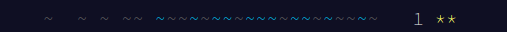## CodeNewbie Community 🌱

Xavier Jouvenot

Posted on • Originally published at 10xlearner.com on

# Advent Of Code 2021 – Sonar Sweep – Puzzle 1

Hello ! I’m Xavier Jouvenot and here is the 1st part of a long series on Advent Of Code 2021.

For this new post, we are going to solve the problem from the 1th December 2021, named "Sonar Sweep". The solution I will propose in C++, but the reasoning can be applied to other languages.

Self promotion: You can find other articles on computer science and programming on my website 😉

## Part 1

### The Problem

The full version of this problem can be found directly on the Advent of Code website, I will only describe the essence of the problem here:

Today, we are looking for the sleigh keys in the ocean, since one of the Elves accidentally sent them into the water. So we jump in Santa submarine (because, of course he has one ! 😄) and we go down the ocean looking for the keys. As the submarine sink into the depth of the ocean, the sonar gives us a report (your puzzle input) in which each line is a measurement of the sea floor depth as the sweep looks further and further away from the submarine, something like:

``````199
200
208
210
200
207
240
269
260
263
``````

Out first mission of the year, is to count the number of times a depth measurement increases from the previous measurement, in order to know what we are dealing with, in the vastness of the ocean.

### Solution

So basically, in plain English, the solution is to iterate through the input (which is an array of numbers), and check if the previous number is bigger than the current one. In C++, it looks like that:

``````#include <array>
#include <iostream>
#include <optional>

constexpr std::array<int, 2000> input = { /* ... */ };

int main()
{
std::optional<int> previousNumber;
size_t numberOfIncrements{0};
for(const auto number : input)
{
if(not previousNumber.has_value()) { previousNumber = number; continue; }
if(*previousNumber < number) { ++numberOfIncrements; }
previousNumber = number;
}

std::cout << "The solution is: " << numberOfIncrements << std::endl;
return 0;
}
``````

First, we define some variables that we are going to need:

``````constexpr std::array<int, 2000> input = { /* ... */ }; // the input of the puzzle
std::optional<int> previousNumber; // variable we are going to use to store the previous number of the array, to be able to compare to it
size_t numberOfIncrements{0}; // number of increments we found so far (the solution we are going to find to the problem)
``````

Then, we iterate through the input, with a range-based for loop:

``````for(const auto number : input) { /* ... */ }
``````

While iterating though the array of inputs, we first check if this is the first number, in which case, there are no previous number to compare about. If this is the case, we update the variable `previousNumber` with the current one, before moving to the next element in the array. In the other case, we compare the previous number of the array to the current one, and if we identify a increase in value, then, we update our result variable `numberOfIncrements` before updating the variable `previousNumber` and moving to the next element in the array.

``````if(not previousNumber.has_value()) { previousNumber = number; continue; }
if(*previousNumber < number) { ++numberOfIncrements; }
previousNumber = number;
``````

Finally, once we have iterate through all the input, we display the result we found in the console:

``````std::cout << "The solution is: " << numberOfIncrements << std::endl;
``````

Spoiler

And, without a surprise, after the Part 1 comes the Part 2 , as, for each day, there are actually 2 problems to solve, the second one being unlocked once the first one is solved.

## Part 2

### Problem

Apparently our previous calculation didn’t give us some useful information, and it would be more useful to consider sums of a three-measurement sliding window, and look for the number of times the sum of measurements in this sliding window increases from the previous sum.

``````199 A
200 A B
208 A B C
210 B C D
200 E C D
207 E F D
240 E F G
269 F G H
260 G H
263 H
``````

In this example, we would compare the sum of the elements marked `A` to the ones marked `B`, and see if the second one is bigger than the first one. If this is the case, we add count the number of increasing values, and go to compare the elements marked `B` to the ones marked `C`, and so on.

### Solution

By adapting the solution to the previous part, we can easily find the solution to this one ! The code for this part looks like:

``````#include <array>
#include <iostream>
#include <optional>

constexpr std::array<int, 2000> input = { /* ... */ };

int main()
{
std::optional<int> previousSum;
size_t numberOfIncrements{0};
for(auto index = 0; index <= input.size() - 3 ; ++index)
{
const auto sum = input[index] + input[index + 1] + input[index + 2];
if(not previousSum.has_value()) { previousSum = sum; continue; }
if(*previousSum < sum) { ++numberOfIncrements; }
previousSum = sum;
}

std::cout << "The solution is: " << numberOfIncrements << std::endl;
return 0;
}
``````

As you can see, I have done a few renaming, so that the variable match what they contain, and instead of iterating through all the array and comparing directly the number in it, we stop iterating before the end, and calculate the sum that we use to compare:

``````for(auto index = 0; index <= input.size() - 3 ; ++index)
{
const auto sum = input[index] + input[index + 1] + input[index + 2];
/* ... */
}
``````

The rest of the code has the same logic has the one of the previous part, so we won’t go into detail about it

Spoiler